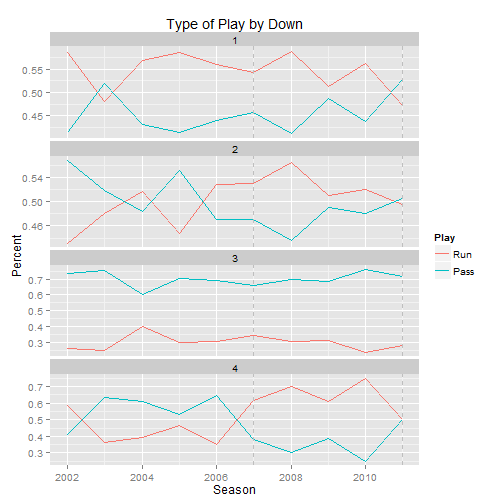January 23, 2013

# Diamonds

``````require(ggplot2)
data(diamonds)
``````  carat       cut color clarity depth table price    x    y    z
1  0.23     Ideal     E     SI2  61.5    55   326 3.95 3.98 2.43
2  0.21   Premium     E     SI1  59.8    61   326 3.89 3.84 2.31
3  0.23      Good     E     VS1  56.9    65   327 4.05 4.07 2.31
4  0.29   Premium     I     VS2  62.4    58   334 4.20 4.23 2.63
5  0.31      Good     J     SI2  63.3    58   335 4.34 4.35 2.75
6  0.24 Very Good     J    VVS2  62.8    57   336 3.94 3.96 2.48``````

# Football

``````load("~/football/data/allGames.rdata")
``````  X1          gameid qtr min sec off def down togo ydline offscore
1  1 20020905_SF@NYG   1  NA   0  SF NYG   NA   NA     NA        0
2  1 20020905_SF@NYG   1  60   0 NYG  SF    1   10     80        0
3  1 20020905_SF@NYG   1  59  25 NYG  SF    2    6     76        0
4  1 20020905_SF@NYG   1  59  20 NYG  SF    3    6     76        0
5  1 20020905_SF@NYG   1  59  20 NYG  SF    3   11     81        0
6  1 20020905_SF@NYG   1  59   9  SF NYG    1   10     23        0
defscore season
1        0   2002
2        0   2002
3        0   2002
4        0   2002
5        0   2002
6        0   2002``````

# Game Descriptions

``head(allGames\$description, 4)``
`````` "J.Cortez kicks 75 yards from SF 30 to NYG -5. R.Dixon  Touchback."
 "(15:00) T.Barber left end to NYG 24 for 4 yards (C.Okeafor  J.Webster)."
 "(14:25) K.Collins pass incomplete to J.Shockey (D.Smith)."
 "(14:20) PENALTY on NYG-J.Shockey  False Start  5 yards  enforced at NYG 24 - No Play."``````

# Histogram

``````hist(diamonds\$carat)
hist(diamonds\$price)``````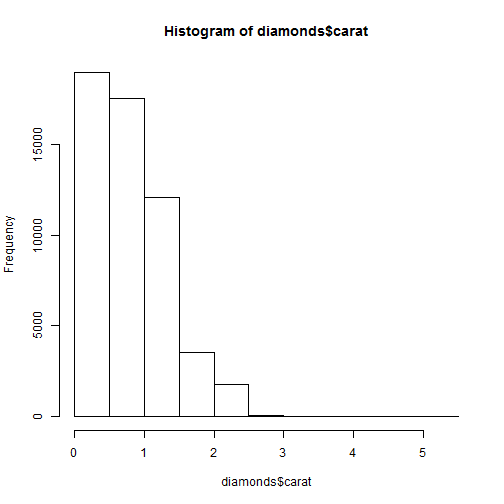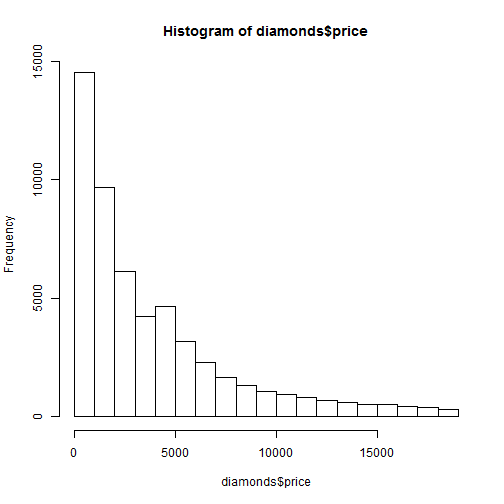# Scatterplot

``plot(price ~ carat, data = diamonds)``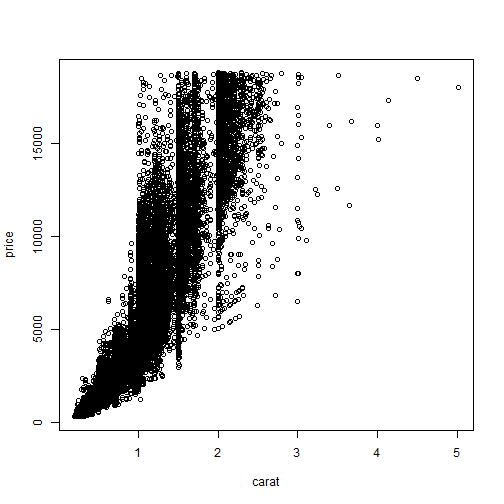# Boxplot

``boxplot(diamonds\$carat)``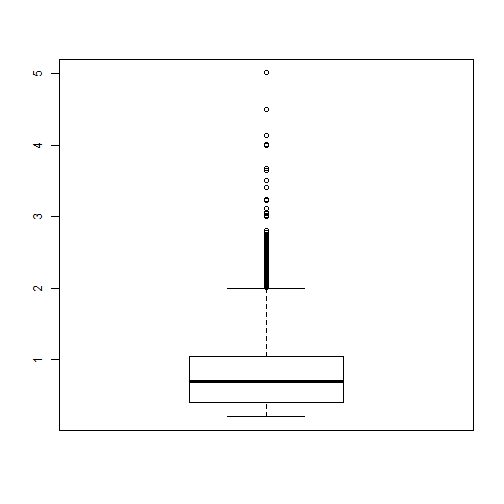# Boxplot Groups

``boxplot(carat ~ cut, data = diamonds)``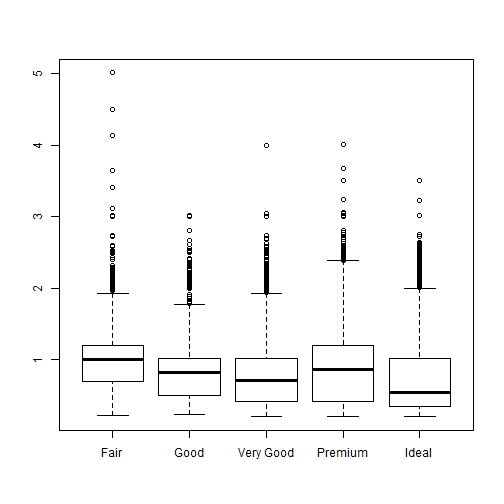# Histogram

``````ggplot(diamonds, aes(x = carat)) + geom_histogram()
ggplot(diamonds, aes(x = price)) + geom_histogram()``````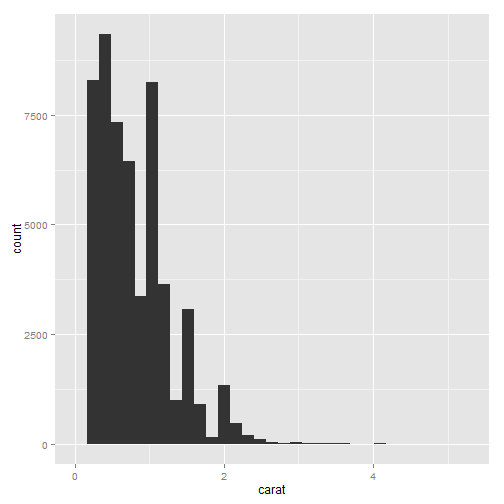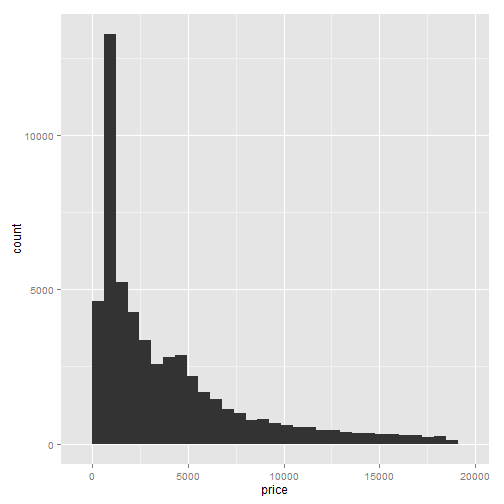# Density

``````ggplot(diamonds, aes(x = price)) + geom_density(fill = "grey50", color = "grey50")
ggplot(diamonds, aes(x = price)) + geom_density(aes(color = cut, fill = cut),
alpha = 1/2)``````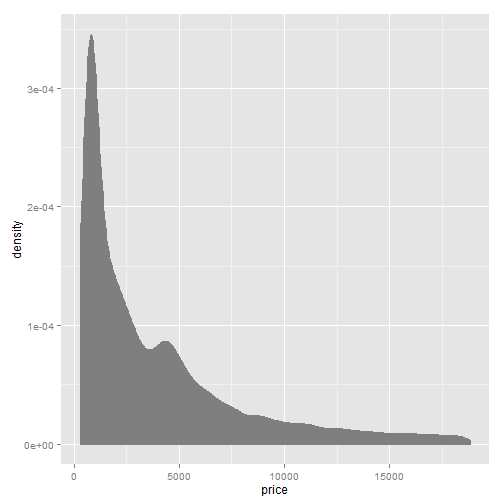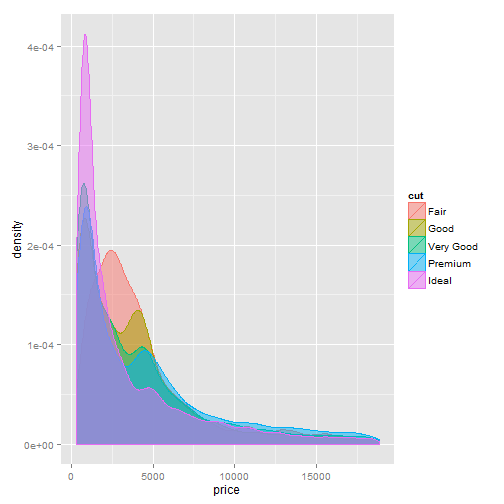# Scatterplot

``````p <- ggplot(diamonds, aes(x = carat, y = price))
p + geom_point()``````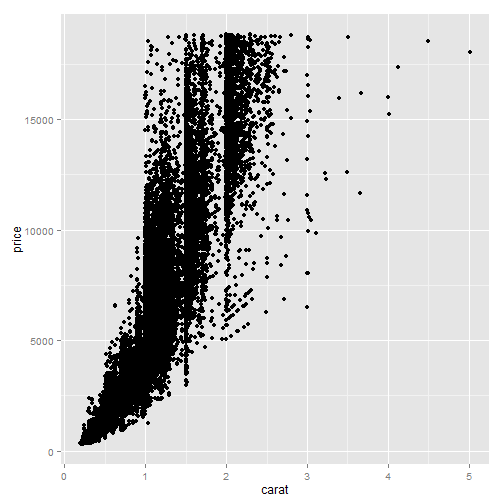# Log Transformation

``ggplot(diamonds, aes(x = log(carat), y = log(price))) + geom_point()``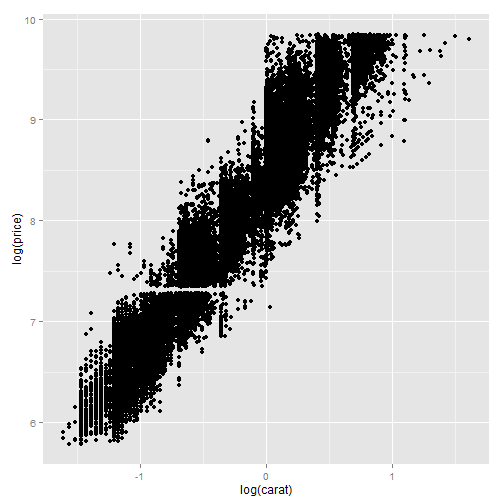# Scatter with Color

``p + geom_point(aes(color = color))``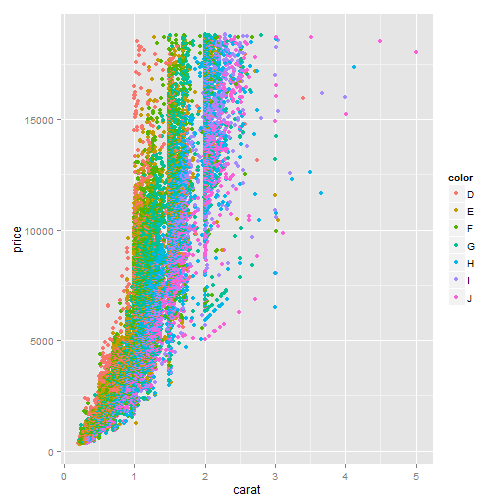# Scatter Wrapping

``p + geom_point(aes(color = color)) + facet_wrap(~cut)``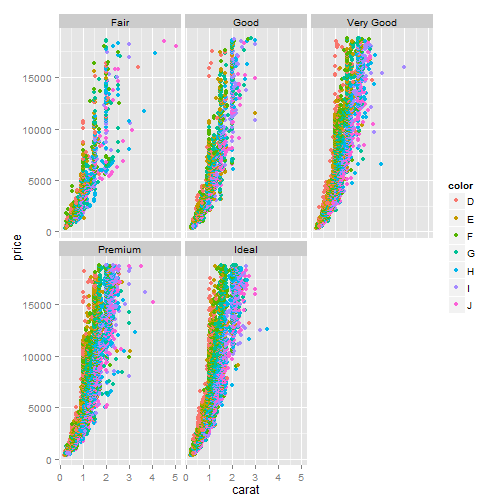# Scatter Wrapping

``p + geom_point(aes(color = color)) + facet_grid(clarity ~ cut)``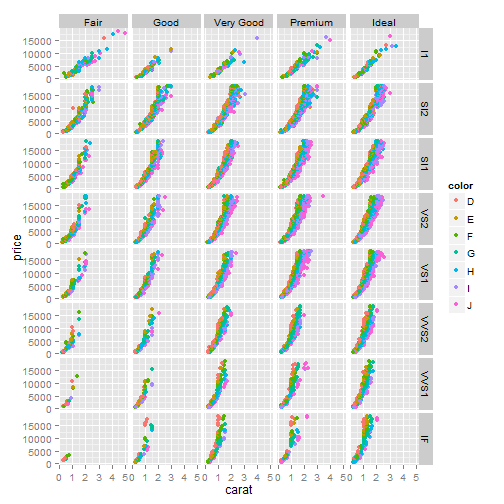# Boxplots

``ggplot(diamonds, aes(y = price, x = 1)) + geom_boxplot()``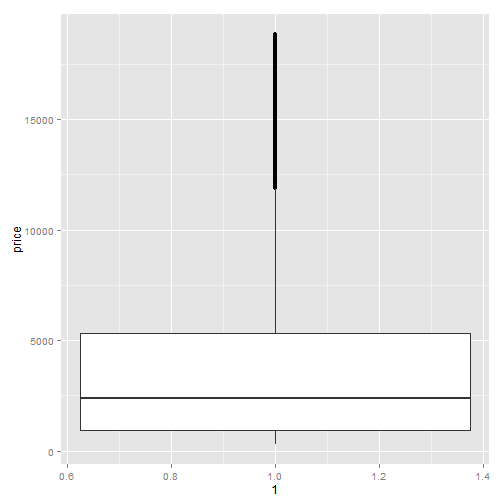# Boxplot by Color

``ggplot(diamonds, aes(y = price, x = cut)) + geom_boxplot()``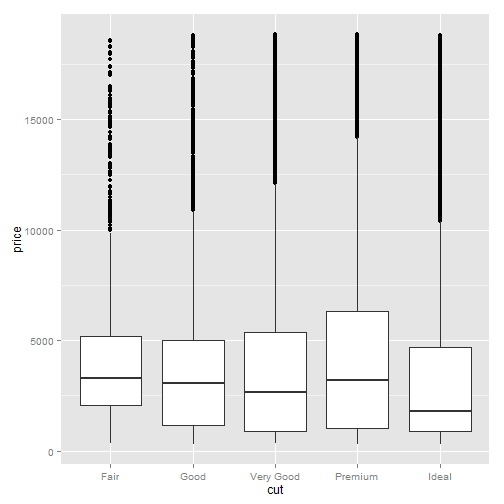# Violin Plot

``````ggplot(diamonds, aes(y = price, x = cut)) + geom_violin()
ggplot(diamonds, aes(y = price, x = cut)) + geom_point() + geom_violin()``````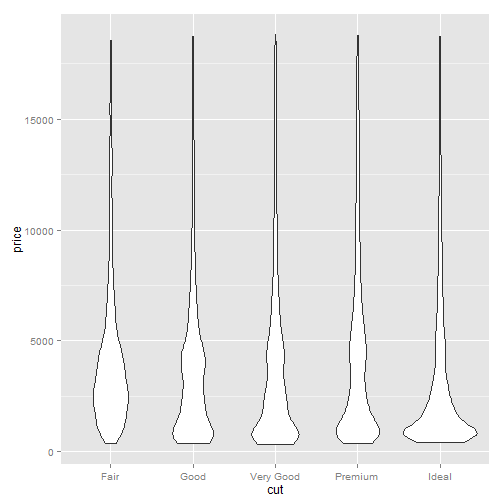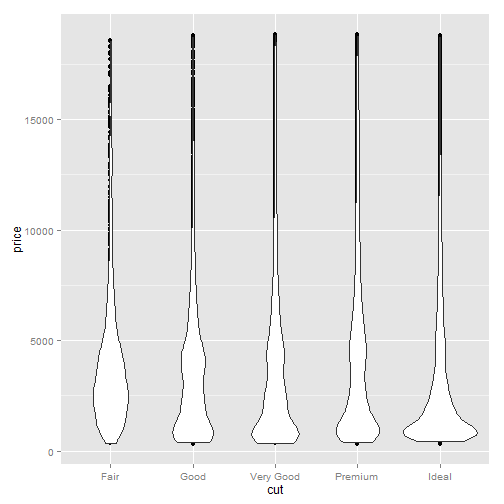# Reshaped Football Data

``````load("~/football/data/playMelt.rdata")
``````  season down Play Percent
1   2002    1 Pass  0.4134
2   2003    1 Pass  0.5186
3   2004    1 Pass  0.4302
4   2005    1 Pass  0.4135
5   2006    1 Pass  0.4391
6   2007    1 Pass  0.4558``````
``tail(playMelt)``
``````   season down Play Percent
75   2006    4  Run  0.3529
76   2007    4  Run  0.6190
77   2008    4  Run  0.7000
78   2009    4  Run  0.6111
79   2010    4  Run  0.7500
80   2011    4  Run  0.5000``````

# Play Selection

``````ggplot(playMelt, aes(x = season, y = Percent, group = Play, color = Play)) +
geom_line() + facet_wrap(~down, ncol = 1, scales = "free_y") + ggtitle("Type of Play by Down") +
labs(x = "Season") + geom_vline(xintercept = c(2007, 2011), color = "grey",
linetype = 2)``````